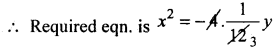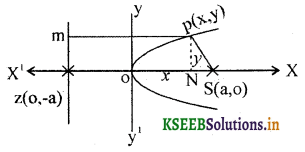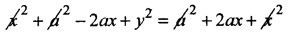# 2nd PUC Basic Maths Question Bank Chapter 16 Parabola

Students can Download Basic Maths Question Bank Chapter 16 Parabola Questions and Answers, Notes Pdf, 2nd PUC Basic Maths Question Bank with Answers helps you to revise the complete Karnataka State Board Syllabus and to clear all their doubts, score well in final exams.

## Karnataka 2nd PUC Basic Maths Question Bank Chapter 16 Parabola

### 2nd PUC Basic Maths Parabola One Mark Questions and Answers

Question 1.
Find the equation of the parabola given that its.
(a) Vertex is (0, 0) and focus is (4, 0)
The curve turn right side with a = 4
∴ Equation of parabola is y2 = 16x

(b) Vertex is (0, 0) and directrix is y = – 3
The curve turn upwords with a = 3
∴ Equation is x2 = 4ay = 4.3y =12y
x2 = 12y

(c) Focus is (1, 0) and the directrix is x = – 1
The curve turns right side with a = 1
And its equation is y2 = 4x

(d) Focus is (-4, 0) and directrix is x = 4
The curve turns left side and a = 4
The equation is y2 = – 16x

(e) Focus is (0, -3) and directrix is y = 3
The curve turns down words and its
Equation is x2 = -12y

(f) Focus is (0, 6) and vertex is (0, 0)
The curve turn upwords and a = 6 its
Equation is x2 = 24y.Question 2.
Find the latus rectum of the parabola y2 = 16x.
Length of L.R = 4a = 16.

Question 3.
Find the focus of the parabola y2 = -8x.
Comparing with y2 = -4ax we get
a = 2, ∴ Focus – (-a, 0) = (-2, 0).

Question 4.
Find the equation of the directrix of the parabolay2 = 8x.
Comparing with y2 = 4ax, 4a = 8 ⇒ a = 2
∴ Equation of directrix x = – 2.

Question 5.
Find the directrix of the parabola 3x2 = -8y.
x2 = $$\frac{-8}{3} y$$
Comparing with x2 = -4ay
4a = $$\frac{8}{3}$$ ⇒ a = $$\frac{2}{3}$$
Equation of dinectrix is y = $$\frac{2}{3}$$ or 3y – 2 = 0.

Question 6.
In the parabola y2 = 8kx, if the length of L.R. is 4 find k.
Comparing with y2 = 4ax we get 4a = 8k
But 4a = 4 (given) ⇒ 4 = 8k
⇒ k = $$\frac { 1 }{ 2 }$$Question 7.
Find the length of L.R of 4y2 + 16x = 0.
Given y2 = -4x
Comparing with y2 = 4ax we get 4a =4
∴ Length of L.R. = 4.

Question 8.
If the length of the L.R. of x2 = 4ky Is 8 find k.
Comparing with x2 = 4ay
4a = 4k
8 = 4k ⇒ k = 2.

Question 9.
Find the length of the L.R of 3x2 + 4y = 0.
x2 = $$\frac { -4 }{ 2 }y$$
Comparing with x2 = -4ay
We get length of L.R. = 4a = $$\frac { 4 }{ 3 }$$

Question 10.
Find the focus of the parabola x2 + 16y = 0.
x2 = 16y
Comparing x2 = -4ay
⇒ 4a = 16 ⇒ a = 4
∴ focus = S(0, -4).

Question 11.
Find the co-ordinates of focus and length of L.R of x4 + 4y = 0.
x2 = -4y
Comparing x2 – 4ay ⇒ 4a = 4 ⇒ a = 1
LT. of L.R = 4, and focus = (0, – 1).Question 12.
Find focus and LT of L.R if y2 = 12x.
Comparing with y2 = 4ax
We get 4a = 12 ⇒ a = 3
∴ Lt of L.R= 12 and focus = (3, 0).

Question 13.
Find the equation of the parabola given that its vertex (0,0), axis is y-axis and passers through $$\left(\frac{1}{2}, 2\right)$$
Given V = (0, 0) and axis is y-axis
∴ The parabola is of the form x2 = 4ay or x2 = -4ay
But this equation passes through the point $$\left(\frac{1}{2}, 2\right)$$
∴$$\left(\frac{1}{2}\right)^{2}$$ = 4a.2 ⇒ $$\frac { 1 }{ 4 }$$ = 8a a = $$\frac { 1 }{ 32 }$$
∴ Required equation of the parabola is x2 = $$\frac { 4 }{ 32 }y$$
x2 = $$\frac { 1 }{ 8 }y$$
8x2 = y = 0.

Question 14.
Find the equation of the parabola with vertex at (0, 0), axis is y-axis and it passes through (-1,-3).
Given V = (0, 0) and axis is y-axis,
∴ The parabola is of the form x2 = 4ay or x2 = 4ay,
But this equation passes through (-1, -3) which ¡s in III quadrant
∴ The curve turn down words and is of the fonn
x2 = -4ay, it passes through (-1, -3)
∴ (-1)2 = 4a(-3) ⇒ 1 = 12a ⇒ a = $$\frac { 1 }{ 12 }y$$x2 = $$\frac { -1 }{ 3 }$$ or 3x2 + y = 0### 2nd PUC Basic Maths Parabola Three or Four Marks Questions and Answers

Question 1.
Write the characteristics of the following.
(i) y2 = 16x
Comparing with y2 = 4ax we get 4a = 16 ⇒ a = 4
Vertex = V(0, 0)
Focus = S(4, 0)
Directrix is x = -4 or x + 4 = 0
Axis x-axis and y = 0
Tangent is y-axis and x = 0
latus rectum is x = 4 or x – 4 = 0
Ends of L.R L (4, 8) L'(4, -8)
Length of L.R = 4a = 4.4 = 16.

Question 2.
y2 + 4x = 0.
y2 = -4x
Comparing this with yy2 = -4ax ⇒ 4a = + 4 ⇒ a = 1
Vertex = (0, 0), focus = S(-1, 0), Directrix is x = 1,or x – 1 = 0
Axis x-axis and y = 0, Tangent is y-axis and x = 0
Ends of L.R, L = (-1, 2) L = (-1; -2)
Equation of L.R is x = – 1 or x + 1 = 0
Length of L.R = 4.Question 3.
x2 = 8y
Comparing this with x2 = 4ay we get 4a = 8 ⇒ a = 2
Vertex = V(0, 0)
Focus = S(0, 2)
Directrix isy = -2 or y + 2 = 0
Axis is y-axis and x = 0,
Tangent is x-axis and y = 0
Ends of L.R., L(4, 2) and L'(-4, 2)
Equation of L.R is y = 2 or y – 2 = 0
Length of L.R = 8.

Question 4.
3x2 = -8y.
⇒ x2 = $$\frac{-8}{3} y$$
Companng with x2 = -4ay we get 4a = $$\frac{+8}{3}$$ ⇒ a = $$\frac{2}{3}$$
Vertex V (0, 0)
Focus = s$$\left(0, \frac{-2}{3}\right)$$
Directrix is y = or 3x – 2 = 0
Axis ¡s y-axis and x = 0
Tangent is x-axis and y = 0
Ends of L.R. L = $$\left(\frac{4}{3}, \frac{-2}{3}\right)$$ and L’= $$\left(\frac{-4}{3}, \frac{-2}{3}\right)$$
EquationofL.R. ¡s y = $$\frac{-2}{3}$$ = 3y + 2 = 0
Length of L.R. = $$\frac{8}{3}$$

Question 5.
Find the equation of a parabola with proper choice of co-ordinate axis is y2 = 4ax.
Proof: Let S be the focus and l be the directrix.
Let SZ = 2 a
⇒ OZ = OS = a
Choose O as the origin and the line ZOX as x-axis and the line YOY’ through O as y-axis. With this choice of co-ordinate axes we have S(a, o) and Z(-a, o)
The equation of directrix is x = – a
Let P(x, y) be any point on the parabola then by the defmation of parabola we have
Distance of P from S = Distance of P fram the line li.e. PS = PM ∵ PM = ZN
PS2 = ZN2
PS2 = (OZ + ON)2
(x – a)2 + y2 = (a + x)2y2 = 4ax which is the equation of the parabolaQuestion 6.
Find the equation of parabola if the vertex and axis of parabola are given.
(a) Vertex is at the orgin and axis along x-aixs and passing through the point p(2,3)
Given vertex = (0, 0) and x-axis So its equation is either y2 = 4ax or y2 = – 4ax, but the
parabola passes through the point P(2, 3) which’ is in 1st quadrant
∴ The parabola turns right side i.e., y2 = 4ax
It passes through (2, 3) ∴ 9 = 4a.2 ⇒ a = $$\frac{9}{8}$$
∴ Equation of parabola is y2 = $$4 . \frac{9}{8} x$$
⇒ 2y2 = 9 x.

(b) Vertex at the origin and passing through the point P(2, -3) and which is symmetric about x-axis.
4 = 12a ⇒ a = $$\frac{1}{3}$$
∴ Equation of parabola is x2 = $$4 . \frac{1}{3} y$$